# Lindelöf theorem

(diff) ← Older revision | Latest revision (diff) | Newer revision → (diff)

on asymptotic values

1) Let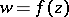be a bounded regular analytic function in the unit disc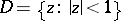and letbe the asymptotic value ofalong a Jordan arcsituated inand ending at a point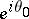, that is,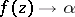as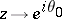along. Thenis the angular boundary value (non-tangential boundary value) ofat, that is,tends uniformly toasinside an angle with vertexformed by two chords of the disc.

The Lindelöf theorem is also true in domainsof other types, and the conditions onhave been significantly weakened. For example, it is sufficient to require thatis a meromorphic function inthat does not assume three different values. Lindelöf's theorem can also be generalized to functionsof several complex variables. For example, ifis a bounded holomorphic function in the ballthat has asymptotic valuealong a non-tangential pathat a point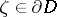, thenis the non-tangential boundary value ofat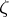(see ).

2) Letbe a bounded regular analytic function in the discthat has asymptotic valuesandalong two distinct pathsandthat end at the point. Thenanduniformly inside the angle between the paths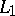and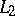. This theorem is also true for domainsof other types. For unbounded functions it is false, generally speaking.

These theorems were discovered by E. Lindelöf .

How to Cite This Entry:
Lindelöf theorem. Encyclopedia of Mathematics. URL: http://encyclopediaofmath.org/index.php?title=Lindel%C3%B6f_theorem&oldid=18228
This article was adapted from an original article by E.D. Solomentsev (originator), which appeared in Encyclopedia of Mathematics - ISBN 1402006098. See original article